Expansion

Chapter 8 Class 11 Binomial Theorem (Deleted)
Concept wise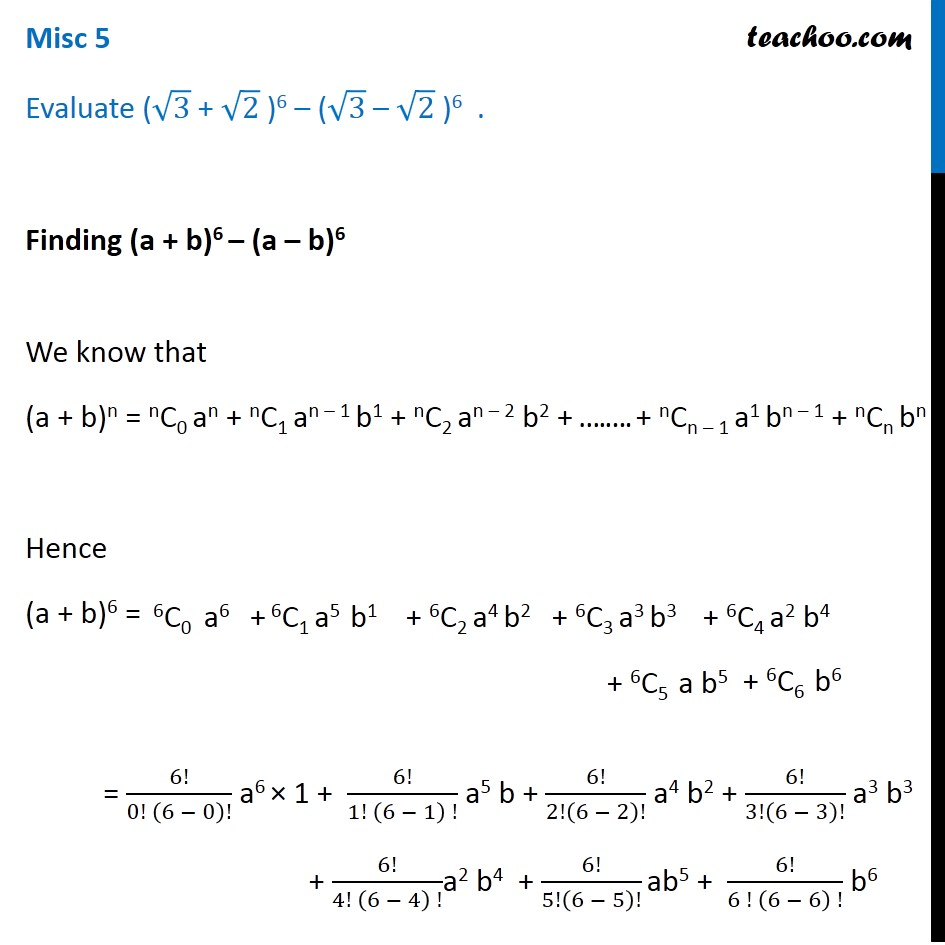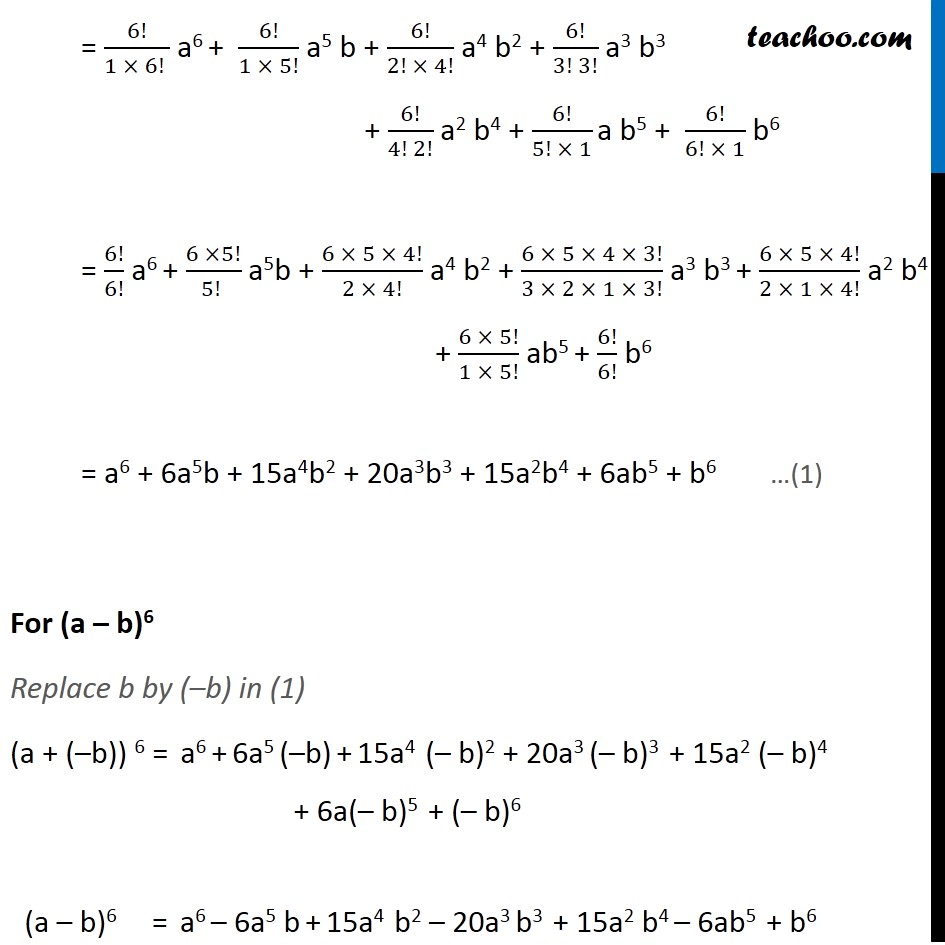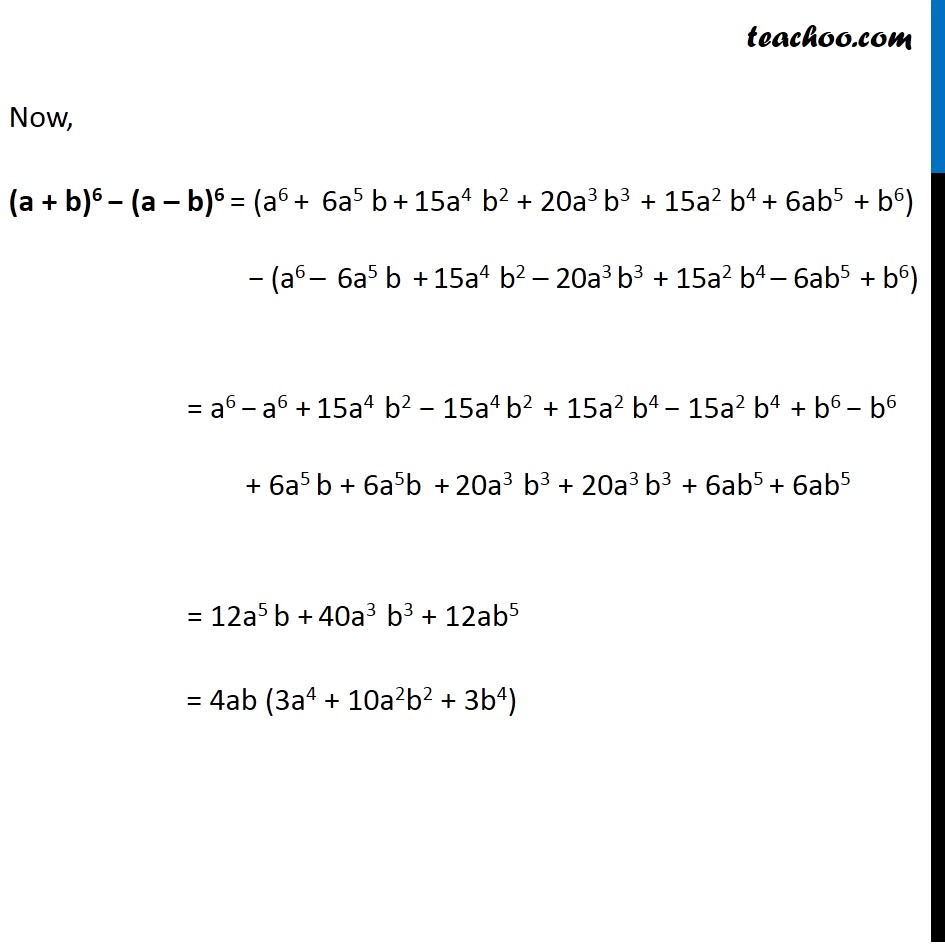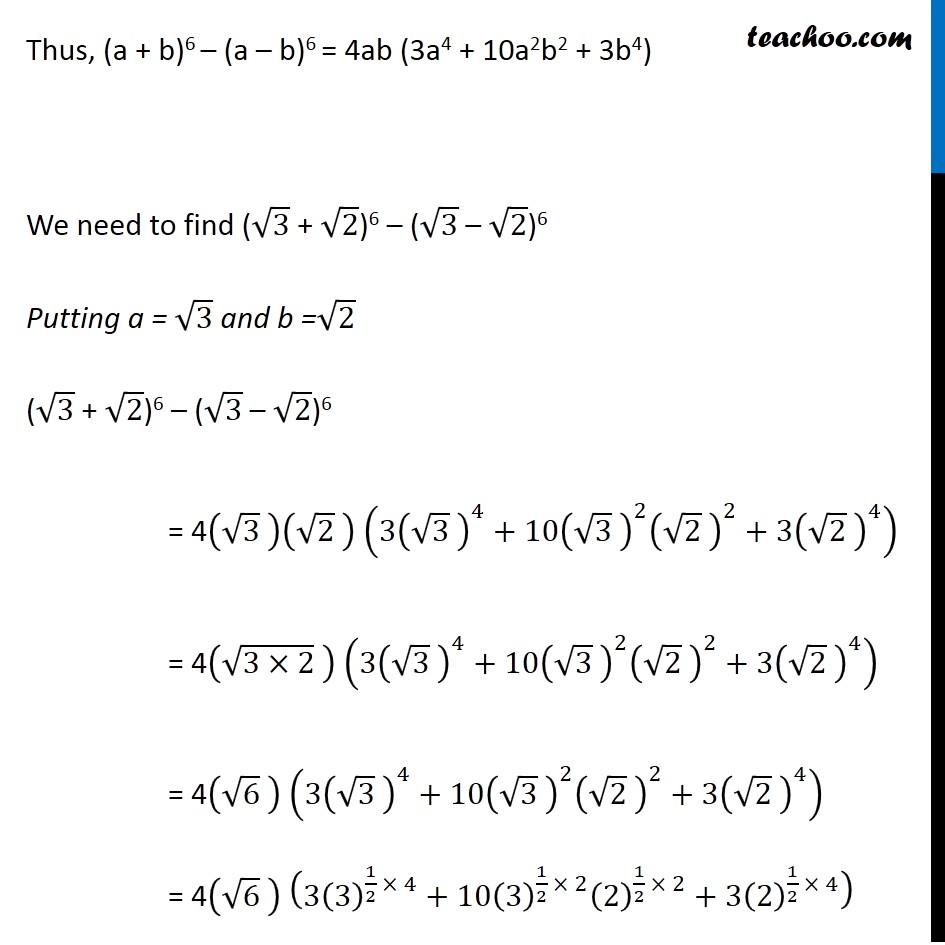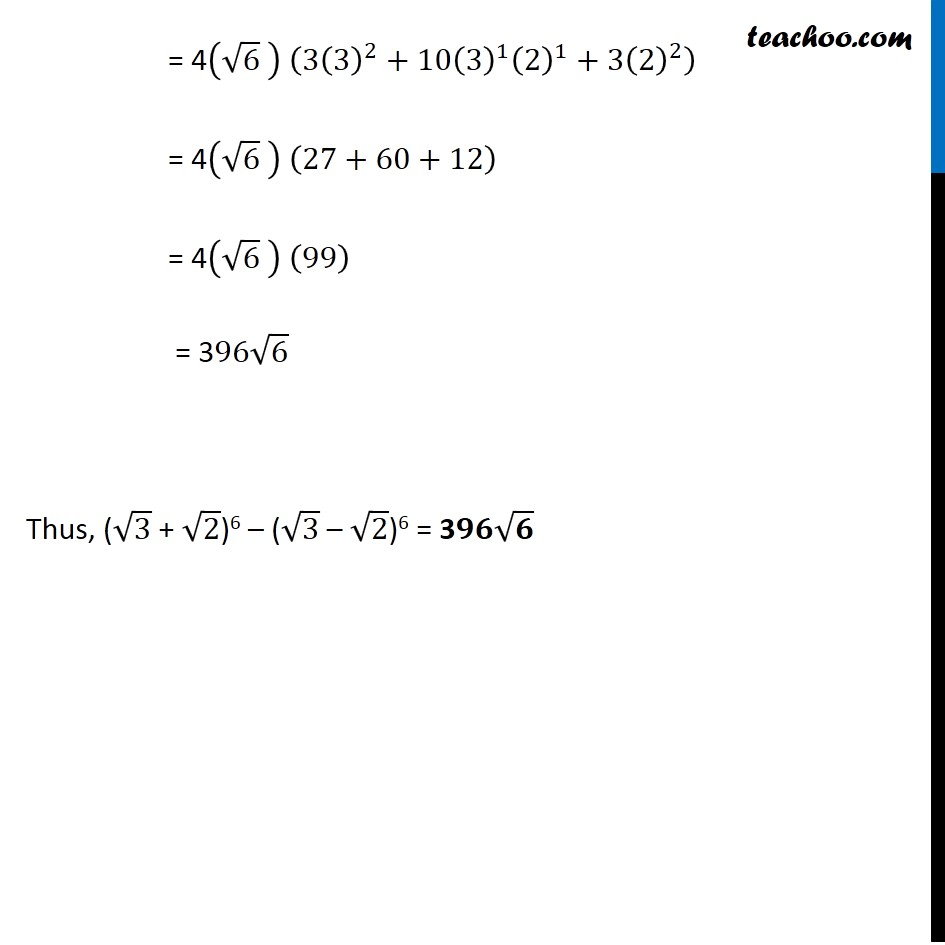### Transcript

Misc 5 Evaluate (√3 + √2 )6 – (√3 – √2 )6 . Finding (a + b)6 – (a – b)6 We know that (a + b)n = nC0 an + nC1 an – 1 b1 + nC2 an – 2 b2 + ….…. + nCn – 1 a1 bn – 1 + nCn bn Hence (a + b)6 = = 6!/(0! (6 − 0)!) a6 × 1 + 6!/(1! (6 − 1) !) a5 b + 6!/2!(6 − 2)! a4 b2 + 6!/3!(6 − 3)! a3 b3 + 6!/(4! (6 − 4) !)a2 b4 + 6!/5!(6 − 5)! ab5 + 6!/(6 ! (6 − 6) !) b6 = 6!/(1 × 6! ) a6 + 6!/(1 × 5!) a5 b + 6!/(2! × 4!) a4 b2 + 6!/(3! 3!) a3 b3 + 6!/(4! 2!) a2 b4 + 6!/(5! × 1) a b5 + 6!/(6! × 1) b6 = 6!/6! a6 + (6 ×5!)/(5! ) a5b + (6 × 5 × 4!)/(2 × 4!) a4 b2 + (6 × 5 × 4 × 3!)/(3 × 2 × 1 × 3!) a3 b3 + (6 × 5 × 4!)/(2 × 1 × 4!) a2 b4 + (6 × 5!)/(1 × 5!) ab5 + 6!/6! b6 = a6 + 6a5b + 15a4b2 + 20a3b3 + 15a2b4 + 6ab5 + b6 For (a – b)6 Replace b by (–b) in (1) (a + (–b)) 6 = a6 + 6a5 (–b) + 15a4 (– b)2 + 20a3 (– b)3 + 15a2 (– b)4 + 6a(– b)5 + (– b)6 (a – b)6 = a6 – 6a5 b + 15a4 b2 – 20a3 b3 + 15a2 b4 – 6ab5 + b6 Now, (a + b)6 − (a – b)6 = (a6 + 6a5 b + 15a4 b2 + 20a3 b3 + 15a2 b4 + 6ab5 + b6) − (a6 – 6a5 b + 15a4 b2 – 20a3 b3 + 15a2 b4 – 6ab5 + b6) = a6 − a6 + 15a4 b2 − 15a4 b2 + 15a2 b4 − 15a2 b4 + b6 − b6 + 6a5 b + 6a5b + 20a3 b3 + 20a3 b3 + 6ab5 + 6ab5 = 12a5 b + 40a3 b3 + 12ab5 = 4ab (3a4 + 10a2b2 + 3b4) Thus, (a + b)6 – (a – b)6 = 4ab (3a4 + 10a2b2 + 3b4) We need to find (√3 + √2)6 – (√3 – √2)6 Putting a = √3 and b =√2 (√3 + √2)6 – (√3 – √2)6 = 4(√3 )(√2 ) (3(√3 )^4+10(√3 )^2 (√2 )^2+3(√2 )^4 ) = 4(√(3×2) ) (3(√3 )^4+10(√3 )^2 (√2 )^2+3(√2 )^4 ) = 4(√6 ) (3(√3 )^4+10(√3 )^2 (√2 )^2+3(√2 )^4 ) = 4(√6 ) (3(3)^(1/2 × 4)+10(3)^(1/2 × 2) (2)^(1/2 × 2)+3(2)^(1/2 × 4) ) = 4(√6 ) (3(3)^2+10(3)^1 (2)^1+3(2)^2 ) = 4(√6 ) (27+60+12) = 4(√6 ) (99) = 396√6 Thus, (√3 + √2)6 – (√3 – √2)6 = 3𝟗𝟔√𝟔